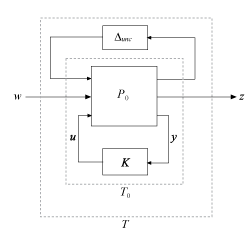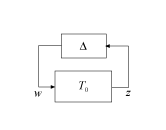## D-K Iteration Process

You can use the `musyn` command to design a robust controller for an uncertain plant, as described in Robust Controller Design Using Mu Synthesis. The algorithm used by `musyn` is an iterative process called D-K iteration. In this process, the function:

1. Uses H synthesis to find a controller that minimizes the closed-loop gain of the nominal system.

2. Performs a robustness analysis to estimate the robust H performance of the closed-loop system. This quantity is expressed as a scaled H norm involving dynamic scalings called the D and G scalings (the D step).

3. Finds a new controller to minimize the scaled H norm obtained in step 2 (the K step).

4. Repeats steps 2 and 3 until the robust performance stops improving.

Both the D step and K step are mathematically intensive computations. Details of the algorithm follow.

### D Step

In the D step, `musyn` computes the upper bound $\overline{\mu }$ of the robust H performance for the current controller K. The D step begins with a robust performance analysis for the closed-loop uncertain system T = LFT(P,K), as in the following diagram.Introducing a performance block Δperf transforms the robust-performance analysis of T to a robust-stability analysis of the feedback loop in the following diagram.Here, Δ is the augmented uncertainty structure

`$\Delta \triangleq \left(\begin{array}{cc}{\Delta }_{perf}& 0\\ 0& {\Delta }_{unc}\end{array}\right).$`

`musyn` computes $\overline{\mu }$, an upper bound on the robust H performance. To do so, `musyn` selects a frequency grid {ω1,…,ωN}. For T with complex uncertainty only, `musyn` computes at each frequency ωi

`${\overline{\mu }}_{i}=\underset{{D}_{i}}{\mathrm{inf}}‖{D}_{i}{T}_{0}\left(j{\omega }_{i}\right){D}_{i}{}^{-1}‖.$`

The frequency-dependent matrices D, which commute with Δ, are called D scalings. $\overline{\mu }$ is the largest result over all frequencies in the grid,

`$\overline{\mu }=\underset{i}{\mathrm{max}}{\overline{\mu }}_{i}.$`

When you use `musyn`, you can access the results of the D step in several ways.

• The default `musyn` display shows $\overline{\mu }$ for each iteration in the `Peak MU` column.

• `musyn` returns $\overline{\mu }$ for each iteration in the `PeakMU` field of the `info` output argument.

• `musyn` returns Di in the `DG` field of the `info` output argument.

• To visualize the frequency-dependence of Di, set the `'Display'` option of `musynOptions` to `'full'`.

For additional details about the computation and interpretation of $\overline{\mu }$, see Robust Performance Measure for Mu Synthesis.

### D-Fitting and Scaled H∞ Performance

`musyn` fits a rational function D(s) to the sequence of scalings {Di}. The fit yields a quantity μF called the scaled H performance,

`${\mu }_{F}\triangleq {‖D\left({T}_{0}\right){D}^{-1}‖}_{\infty }.$`

Because the fit is not exact, μF is typically somewhat larger than $\overline{\mu }$.

You can access the results of the fit in several ways.

• The default `musyn` display shows μF for each iteration in the ```DG Fit``` column.

• `musyn` returns μF for each iteration in the `PeakMUFit` field of the `info` output argument.

• `musyn` returns the fitting functions in the `dr` and `dc` fields of the `info` output argument.

• To visualize the frequency dependence of the fitting functions, set the `'Display'` option of `musynOptions` to `'full'`.

### K Step

T0 depends on the choice of controller K by the relation T0 = LFT(P0,K). Therefore, minimizing μF with respect to K is a scaled H synthesis problem. Thus, in the K step, `musyn` uses `hinfsyn` or `hinfstruct` to compute a controller K* that minimizes μF. The minimized quantity is the scaled H norm. For the algorithm to make progress, the new controller must improve the robust performance obtained in the D step:

`${‖D\text{\hspace{0.17em}}\text{LFT}\left({P}_{0},{K}^{*}\right)\text{\hspace{0.17em}}{D}^{-1}‖}_{\infty }<\overline{\mu }.$`

Otherwise, the progress is not sufficient for compensate for fitting errors. Thus `musyn` terminates D-K iteration process when K* does not improve the robust performance within the tolerance specified by the `'TolPerf'` option of `musynOptions`.

You can access the results of the K step in several ways.

• The default `musyn` display shows the scaled H norm for each iteration in the ```K Step``` column.

• `musyn` returns the new controller in the `K` of the `info` output argument, and the corresponding scaled H norm for each iteration in the `gamma` field.

### Mixed Real and Complex Uncertainty

When the system has both real and complex uncertainty and you set the `'MixedMU'` option of `musynOptions` to `'on'`, `musyn` uses an additional G-scaling to improve the computation of $\overline{\mu }$. The algorithm in this case is called mixed-μ synthesis.

For mixed uncertainty, `musyn` computes ${\overline{\mu }}_{i}$ and scalings Dr(ωi), Dc(ωi), and Gcr(ωi) such that

`${\left(\begin{array}{c}{T}_{0}\left(j{\omega }_{i}\right)\\ I\end{array}\right)}^{H}\left(\begin{array}{cc}{D}_{r}\left({\omega }_{i}\right)& -j{G}_{cr}^{H}\left({\omega }_{i}\right)\\ j{G}_{cr}\left({\omega }_{i}\right)& -{\overline{\mu }}_{i}^{2}{D}_{c}\left({\omega }_{i}\right)\end{array}\right)\left(\begin{array}{c}{T}_{0}\left(j{\omega }_{i}\right)\\ I\end{array}\right)\le 0$`

at each frequency in the grid.

`musyn` fits the D and G scaling data by constructing a rational function

`$F\left(s\right)=\Psi \left(s\right)\left(\begin{array}{cc}{d}_{r}\left(s\right)& 0\\ 0& {d}_{c}\left(s\right)\end{array}\right)$`

such that

• dr(s), dc(s), and Ψ(s) are stable with stable inverse.

• dr(s) and dc(s) approximate the square roots of the diagonal entries of Dr(ωi) and Dc(ωi).

• F approximately satisfies

`$\begin{array}{c}\left(\begin{array}{cc}{D}_{r}\left({\omega }_{i}\right)& -j{G}_{cr}^{H}\left({\omega }_{i}\right)\\ j{G}_{cr}\left({\omega }_{i}\right)& -{\mu }^{2}{D}_{c}\left({\omega }_{i}\right)\end{array}\right)\approx F{\left(j{\omega }_{i}\right)}^{H}J\text{\hspace{0.17em}}F\left(j{\omega }_{i}\right),\\ J=\left(\begin{array}{cc}{I}_{r}& 0\\ 0& -I{}_{c}\end{array}\right).\end{array}$`

Finally, the scaled H performance is defined as

`${\mu }_{F}\triangleq {‖\overline{T}\left(s\right)‖}_{\infty },$`

where $\overline{T}\left(s\right)$ is the transformed system,

`$\overline{T}\left(s\right)\triangleq \overline{\mu }{\overline{T}}_{1}{\overline{T}}_{2}^{-1},\text{ }\left(\begin{array}{c}{\overline{T}}_{1}\left(s\right)\\ {\overline{T}}_{2}\left(s\right)\end{array}\right)\triangleq \Psi \left(s\right)\left(\begin{array}{c}{d}_{r}\left(s\right){T}_{0}\left(s\right)\\ {d}_{c}\left(s\right)\end{array}\right).$`

For an exact fit of D and G, $‖\overline{T}\left(j{\omega }_{i}\right)‖={\overline{\mu }}_{i}$. Therefore, in general, ${\mu }_{F}\ge \overline{\mu }.$

Because the transformed system $\overline{T}\left(s\right)$ is still a linear fractional function of the controller K, the K step for the mixed-μ case proceeds by computing a controller K* that minimizes ${‖\overline{T}‖}_{\infty }$.

When using `musyn`, you can access the D and G scalings in several ways.

• `musyn` returns the D and G scaling data in the `DG` field of the `info` output argument.

• `musyn` returns the fitting functions in the `dr`, `dc`, and `PSI` fields of the `info` output argument.

• To visualize the frequency dependence of the scaling data and fitting functions, set the `'Display'` option of `musynOptions` to `'full'`.